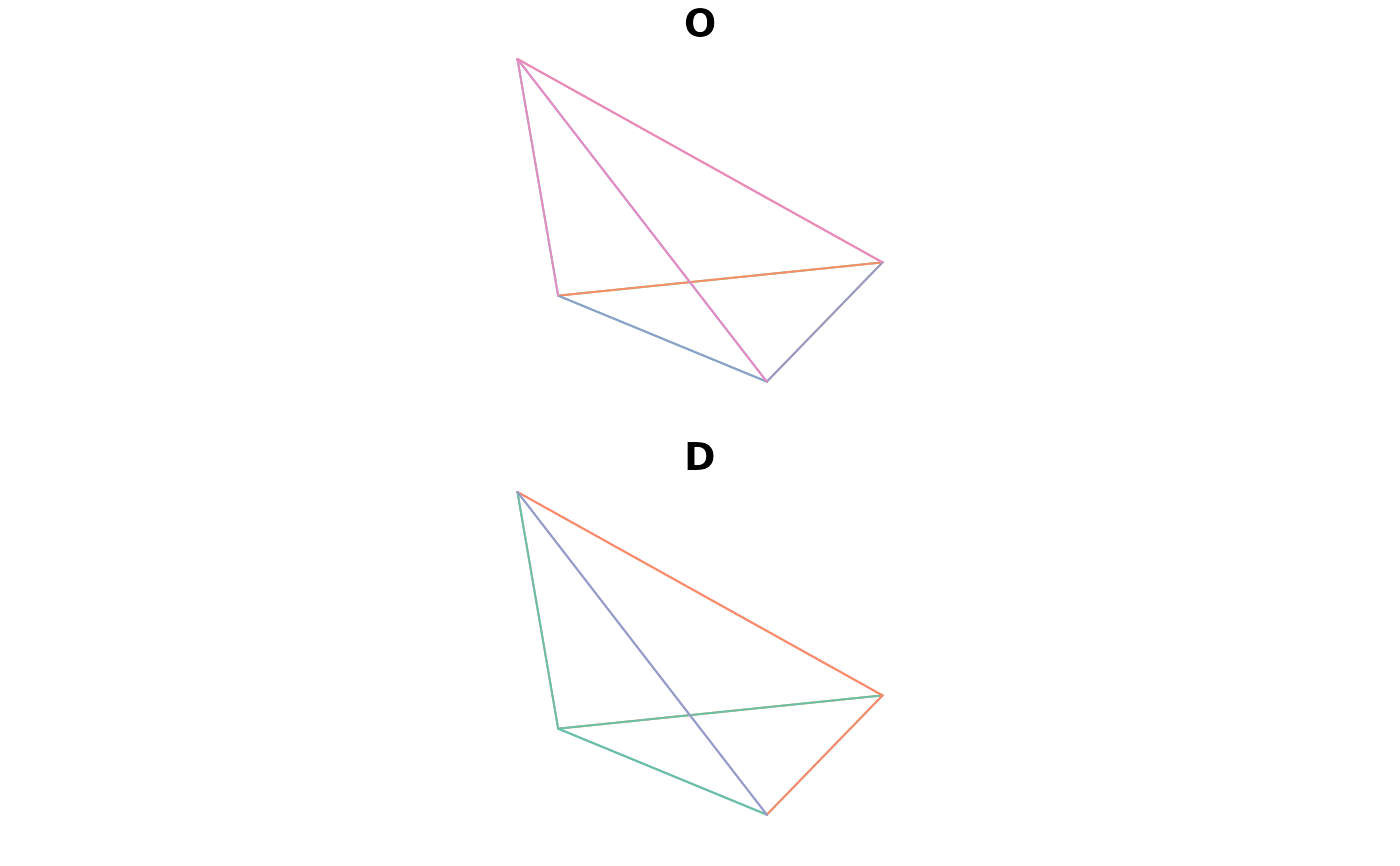Takes a series of geographical points and converts them into a spatial (linestring) object representing the potential flows, or 'spatial interaction', between every combination of points.

## Usage

``points2flow(p)``

## Arguments

p

A spatial (point) object

Other od: `od2line()`, `od2odf()`, `od_aggregate_from()`, `od_aggregate_to()`, `od_coords2line()`, `od_coords()`, `od_id_order()`, `od_id`, `od_oneway()`, `od_to_odmatrix()`, `odmatrix_to_od()`, `points2odf()`
``````flow_sf <- points2flow(cents_sf[1:4, ])